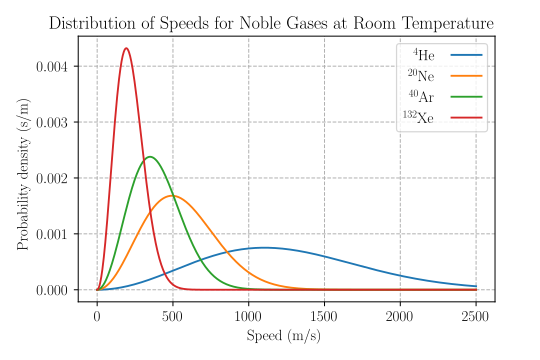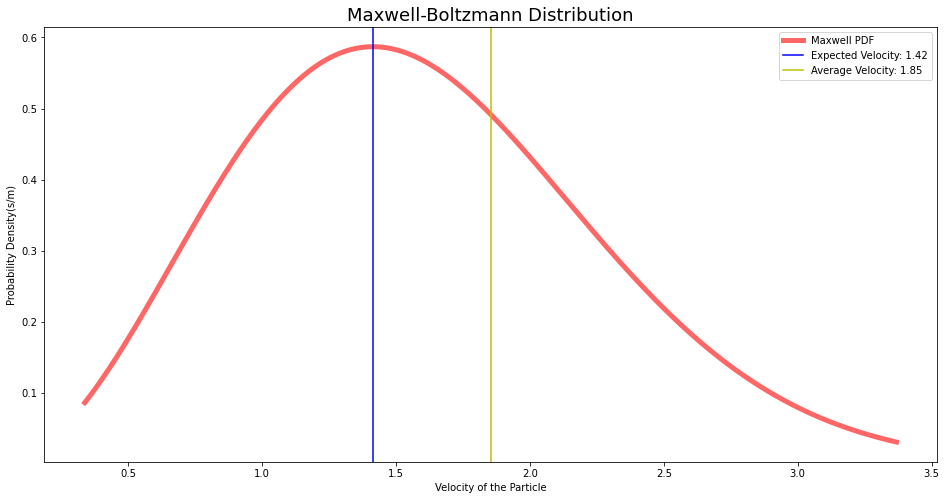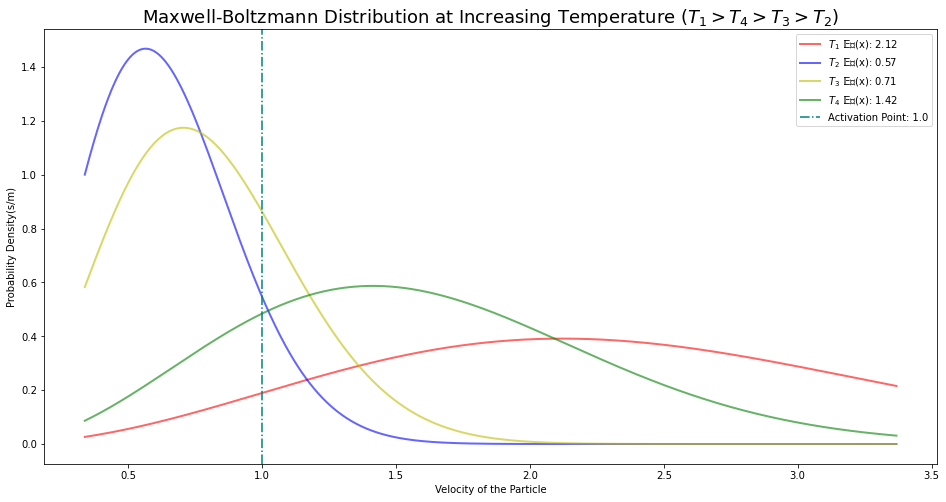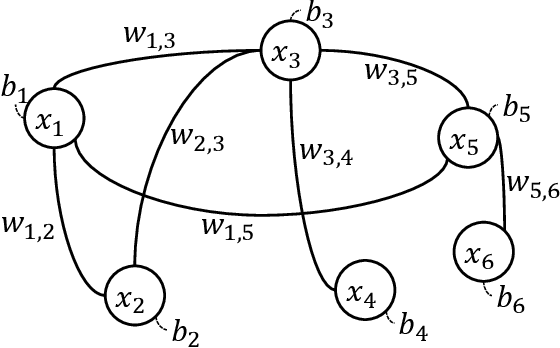### Temperature is Nothing but Measure of Speed of the Particles at Molecular Scale - Intro 2 Maxwell Boltzmann Distribution

Posted January 23, 2022 by Gowri Shankar  ‐  7 min read

The definition for temperature is it is the average kinetic energy of the molecules in the space. If you find the cup of coffee your girlfriend graciously gave you this morning is not hot enough, then you can confidently conclude the molecules in the coffee pot are as lazy as you are. When the particles in the space are active, bumping into each other and have a commotion to prove their existence, we can call they are hot. What makes one hot is directly proportional to the number of particles in their space of influence traipse from a steady-state to a hyperactive one. Often these particles move aimlessly that we witness while boiling water or cooking food. This phenomenon can be understood quite clearly via Maxwell-Boltzmann distribution which is a concept from Statistical Physics/Mechanics having significant importance in machine learning and cognitive science.

In this post, we shall study the concept of Maxwell-Boltzmann distribution which is a foundational concept in machine learning for topics like Boltzmann Machine, Restricted Boltzmann Machine, and Energy-Based Models. This post comes under a new topic Energy-Based Models under Probabilistic ML/DNN. Previous posts under probabilistic machine learning can be found here,### Objective

The objective of the post is to

• Understand Maxwell-Boltzmann Distribution
• Overview of Boltzmann Machine and
• An Introduction to Energy-Based Models

## Introduction

Maxwell-Boltzmann distribution is a probability distribution that describes the particle speed in a container that exhibits momentum and exchange energy in the quest of attaining thermal equilibrium. When we speak about the speed of an entity we often assume it is the velocity of the entity. i.e. amplitude and direction are expected to represent the velocity(a vector). In the case of particle speed, we measure the speed in 3-dimensional space without the direction, hence it is a scalar representation. To be precise, from the container we sample a random particle and measure its speed, then expect the speed to be closer to the average speed of all the particles in the container. Maxwell came up with this idea and Boltzmann improved it significantly to make a foundation that this distribution maximizes the entropy of the container. Entropy is always a dearer topic for the machine learning fraternity to ponder over.


The Maxwell–Boltzmann distribution concerns the distribution of an
amount of energy between identical but distinguishable particles.
It represents the probability for the distribution of the states
in a system having different energies.

- Dario Camuffo, 2019



$$\Large PDF(x) = \sqrt{\frac{2}{\pi}} \frac{x^2e^{-x^2/2 a^2}}{a^3} \tag{1. PDF function of Max-Boltz Dist}$$ $$a = \sqrt{\frac{kT}{m}}$$ Where,

• $k$ is the Boltzmann Constant
• $T$ is the ambient temperature
• $m$ is the mass of the molecule
• $x$ is the velocity of the moleculesTrivia

• If we increase the temperature, the peaks of the noble gases lower and the area under the curve remains the same(because the number of molecules remains the same)
• At room temperature Velocity of particles of $Xenon < Argon < Neon < Helium$
• Probability density decreases exponentially as the velocity increases

An excellent simulation of Maxwell-Boltzmann distribution

### Expected Value vs Mean

We often consider the expected value and the mean are same/similar with certain amount of discomfort. The expected value and mean will be the same(without a doubt) only when the underlying distribution is uniform. It is easy for one to intuitively understand the difference from the particle speed vs temperature analogy that we studied in the previous section. On close observation of the distribution graph of noble gases, we notice the plot is left-skewed for Xeon and not reaching zero density for Helium. i.e. You pick a sample particle from the Xeon container, the speed of the particle is expected to be equal to the speed at the peak probability density. However, this expected speed is less than the average speed of all the particles from the container. As density lowers, the expected speed gets closer and closer to the mean.

from scipy.stats import maxwell
import numpy as np
import matplotlib.pyplot as plt
fig, ax = plt.subplots(1, 1, figsize=(16, 8))

x = np.linspace(maxwell.ppf(0.01), maxwell.ppf(0.99), 1000)
pdf = maxwell.pdf(x)
plt.plot(x, pdf, 'r-', lw=5, alpha=0.6, label='Maxwell PDF')
expected_velocity = x[np.argmax(pdf)]
plt.axvline(x=expected_velocity, color='b', label = f'Expected Velocity: {round(expected_velocity, 2)}')
plt.axvline(x=np.mean(x), color='y', label = f'Average Velocity: {round(np.mean(x), 2)}')
plt.title("Maxwell-Boltzmann Distribution", fontsize=18)
plt.xlabel("Velocity of the Particle")
plt.ylabel("Probability Density(s/m)")
plt.legend()
plt.show()### Activation Energy

The probability distribution of Maxwell-Boltzmann distribution is defined using an Energy function $\mathbb{E}_v(x)$. Thermal energy is a form of kinetic and potential energy, we know the particles in the space have their inherent potential energy. When an external catalyst(or activation is done) is added, assume we are heating the container - pressure increases and increases particle speed. This makes the particles in the right tail of the distribution agitate and get ready for the external reaction. Let us call this energy required as activation energy. i.e. The amount of energy required to make the particles move to a velocity for a chemical reaction is activation energy. For example, at 100 Degrees Celcius water is ready to change its state from liquid to vapor - the energy required for that transition is an example of activation energy.

fig, ax = plt.subplots(1, 1, figsize=(16, 8))

x = np.linspace(maxwell.ppf(0.01), maxwell.ppf(0.99), 1000)
pdf = maxwell.pdf(x, loc=0, scale=1.5)
plt.plot(x, pdf, 'r-', lw=2, alpha=0.6, label=f"$T_1$ 𝔼𝑣(x): {round(x[np.argmax(pdf)], 2)}")

pdf1 = maxwell.pdf(x, loc=0, scale=0.4)
plt.plot(x, pdf1, 'b-', lw=2, alpha=0.6, label=f"$T_2$ 𝔼𝑣(x): {round(x[np.argmax(pdf1)], 2)}")

pdf2 = maxwell.pdf(x, loc=0, scale=0.5)
plt.plot(x, pdf2, 'y-', lw=2, alpha=0.6, label=f"$T_3$ 𝔼𝑣(x): {round(x[np.argmax(pdf2)], 2)}")

pdf3 = maxwell.pdf(x, loc=0, scale=1.0)
plt.plot(x, pdf3, 'g-', lw=2, alpha=0.6, label=f"$T_4$ 𝔼𝑣(x): {round(x[np.argmax(pdf3)], 2)}")

plt.axvline(x=1.0, color='teal', linestyle="dashdot", label = f'Activation Point: {1.0}')
plt.title("Maxwell-Boltzmann Distribution at Increasing Temperature ($T_1 > T_4 > T_3 > T_2$)", fontsize=18)
plt.xlabel("Velocity of the Particle")
plt.ylabel("Probability Density(s/m)")
plt.legend()
plt.show()This plot is to demonstrate, applying energy at a steady interval the expected value shifts lower by renormalizing the probability between $[0, 1]$. When we define a function with all possible states of energy and use it as the objective function for our models, we call them energy-based models.

## Boltzmann Machines


A Boltzmann machine defines a probability distribution over
binary-valued patterns. One can learn parameters of a Boltzmann
machine via gradient based approaches in a way that log
likelihood of data is increased. The gradient and Hessian of a
Boltzmann machine admit beautiful mathematical representations,
although computing them is in general intractable. This
intractability motivates approximate methods, including Gibbs
sampler and contrastive divergence, and tractable alternatives,
namely energy-based models.

- Takayuki Osogami, 2019The energy of a Boltzmann machine is defined by

$$E_{\theta}(x) = - \sum_{i=1}^N b_i x_i - \sum_{i=1}^{N-1} \sum_{j=i+1}^N w_{i,j} x_i x_j$$ $$\Large \mathbb{E}_{\theta}(x) = -b^Tx - x^TWx \tag{1. Energy of a Boltzmann Machine}$$

Where,

• N is the number of units in the Boltzmann machine
• $X_i$ is the random value representing $i^{th}$ unit for $i \in [1, N]$
• $b_i$ is the bias of the $i^{th}$ unit for $i \in [1, N]$
• $w_{i,j}$ is the weight between $i^{th}$ and $j^{th}$ unit for $(i, j) \in [1, N-1] \times [i+1, N$ ]
• The bias $b$ and weight $W$ are defined as $\theta= (b, W)$

Then the probability distribution over binary patterns is, $$\Large P_{\theta}(x) = \frac{e^{-\mathbb{E}_{\theta}(x)}}{\sum_{\tilde{x}} e^{-\mathbb{E}_{\theta}(\tilde{x})}} \tag{2. PDF of Boltzmann Machine}$$

In the Boltzmann machine, some of the units are hidden and some are visible.

• Hidden Units - They are not having any direct influence over the target probability. They represent specific properties that cannot be represented by the visible units
• Visible Units - They influence the target probability. The visible units are divided into input and output units.

The goal of such an architecture is to determine the pattern of target probability distribution $\mathbb{P}_{target}(.$) by optimally setting the values for $\theta$ - makes them generative models.

### Hebbian Properties

Hebb’s rule states neurons wire together fire together. The Boltzmann machine provides a theoretical foundation for Hebb’s rule of learning biological neural networks. Bolt


When an axon of cell A is near enough to excite a cell B
and repeatedly or persistently takes part in firing it,
some growth process or metabolic change takes place in one
or both cells such that A’s efficiency, as one of the cells
firing B, is increased.

- Takayuki Osogami, 2019


• A unit of the Boltzmann machine represents to a neuron
• If the unit $i$ fires, $X_i=1$ it means $i^{th}$ neuron is fired
• Two neurons, $i, j$ fires $\rightarrow X_i(w)X_i(w) = 1$ then $w_{i,j}$ get stronger
• i.e $0 < \mathbb{E}[X_i, X_j] < 1$ for $(i, j)$ neurons for the finite values of $\theta$

The learning rule is derived from a stochastic model with an objective function that minimizes KL divergence to the target distribution or maximizes the log-likelihood of training data.

## Epilogue

We briefly described the Boltzmann machine after learning the inspiration for energy-based models by studying the elegance of Maxwell-Boltzmann Distribution. This post introduces the energy-based models to the reader at a very high level. It also clarifies the idea of expected values intuitively. The goal of this post is to explain the use of energy(funcitons) in deep learning with a non-invasive(not many jargons) approach. We have built a foundation for future learnings that includes,

• Restricted Boltzmann Machine
• Boltzmann machines for time series analysis
• Applications in cognitive sciences etc
# temperature-is-nothing-but-measure-of-speed-of-the-particles-at-molecular-scale-intro-2-maxwell-boltzmann-distribution